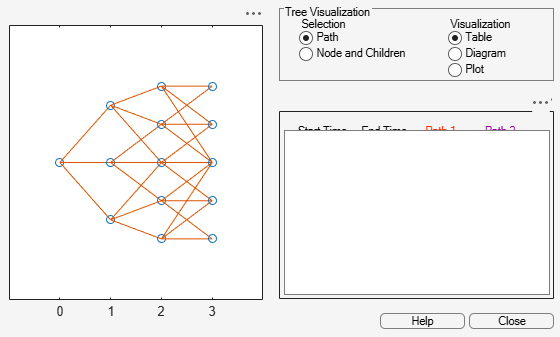cvtree

Convert inverse-discount tree to interest-rate tree

Description

example

RateTree = cvtree(Tree) converts a tree structure using inverse-discount notation to a tree structure using rate notation for forward rates.

Examples

collapse all

Convert a Hull-White tree using inverse-discount notation to a Hull-White tree displaying interest-rate notation.

HWTree
HWTree = struct with fields:
FinObj: 'HWFwdTree'
VolSpec: [1x1 struct]
TimeSpec: [1x1 struct]
RateSpec: [1x1 struct]
tObs: [0 1 2 3]
dObs: [731947 732313 732678 733043]
CFlowT: {[4x1 double]  [3x1 double]  [2x1 double]  }
Probs: {[3x1 double]  [3x3 double]  [3x5 double]}
Connect: {  [2 3 4]  [2 2 3 4 4]}
FwdTree: {1x4 cell}

HWTree.FwdTree{1}
ans = 1.0279
HWTree.FwdTree{2}
ans = 1×3

1.0528    1.0356    1.0186

Use treeviewer to display the path of interest rates expressed in inverse-discount notation.

treeviewer(HWTree)Use cvtree to convert the inverse-discount notation to interest-rate notation.

RTree = cvtree(HWTree)
RTree = struct with fields:
FinObj: 'HWRateTree'
VolSpec: [1x1 struct]
TimeSpec: [1x1 struct]
RateSpec: [1x1 struct]
tObs: [0 1 2 3]
dObs: [731947 732313 732678 733043]
CFlowT: {[4x1 double]  [3x1 double]  [2x1 double]  }
Probs: {[3x1 double]  [3x3 double]  [3x5 double]}
Connect: {  [2 3 4]  [2 2 3 4 4]}
RateTree: {1x4 cell}

RTree.RateTree{1}
ans = 0.0275
RTree.RateTree{2}
ans = 1×3

0.0514    0.0349    0.0185

use treeviewer to display the converted tree, showing the path of interest rates expressed as forward rates.

treeviewer(RTree)Input Arguments

collapse all

Tree structure, specified by Heath-Jarrow-Morton, Black-Derman-Toy, Hull-White, Black-Karasinski, or Cox-Ingersoll-Ross tree structure that uses inverse-discount notation for forward rates.

Data Types: struct

Output Arguments

collapse all

Tree structure using rate notation for forward rates, returned as a tree structure.